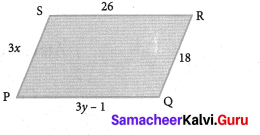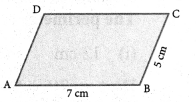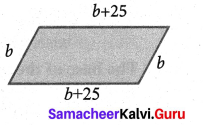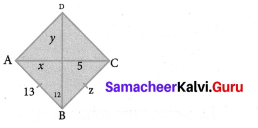# Samacheer Kalvi 7th Maths Solutions Term 1 Chapter 2 Measurements Additional Questions

Students can Download Maths Chapter 2 Measurements Additional Questions and Answers, Notes Pdf, Samacheer Kalvi 7th Maths Book Solutions Guide Pdf helps you to revise the complete Tamilnadu State Board New Syllabus and score more marks in your examinations.

## Tamilnadu Samacheer Kalvi 7th Maths Solutions Term 1 Chapter 2 Measurements Additional Questions

Exercise 2.1

Question 1.
In the following figure, PQRS is a parallelogram find x and y.Solution:
We know that in a parallelogram opposite sides are equal.
∴ 3x = 18
x = $$\frac{18}{3}$$
x = 6 and
3y – 1 = 26
3y = 26 + 1 = 27
y = $$\frac{27}{9}$$
y = 9

Question 2.
Two adjacent sides of a parallelogram are 5 cm and 7 cm respectively. Find its perimeter.
Solution:Perimeter = AB + BC + CD + AD [∵ AB = DC & AD = BC]
= 7 cm + 5 cm + 7 cm + 5 cm = 24 cm

Question 3.
The perimeter of a parallelogram is 150 cm. One of its sides is greater than the other by 25 cm. Find the length of the sides of the parallelogram.
Solution:Given perimeter = 150 cm
Let one side of the parallelogram be ‘b’ cm
Then the other side = b + 25 cm
b + (b + 25) + b + (b + 25) = 150
b + b + 25 + b + b + 25 = 150
4h + 50 = 150 =4b = 100
b = $$\frac{100}{4}$$ = 25
∴ One side b = 25 cm
Other side b + 25 = 50 cmExercise 2.2

Question 1.
ABCD is a rhombus. Find x, y, and z.Solution:
We know that all sides of rhombus are equal and its diagonals bisect each other.
∴ x = 5, y = 12 and z = 13.

Question 2.
Find the altitude of the rhombus whose area is 315 cm2 and its perimeter is 180 cm.
Solution:
Given perimeter of the rhombus = 180 cm
∴ One side of the rhombus = $$\frac{180}{4}$$ = 45 cm
Given area of the rhombus = 315 cm2
b × h = 315
45 × h = 315 = $$\frac{315}{45}$$
h = 7 cm
Altitude of the rhombus = 7 cm

Question 3.
The floor of a building consists of 2000 titles which are rhombus shaped and each of its diagonals are 40 cm and 25 cm. Find the total cost of polishing the floor, if the cost per m2 = ₹ 5.
Solution:
Area of each title = $$\frac{1}{2}$$ × d1 × d2 sq. units
= $$\frac{1}{2}$$ × 40 × 25 cm2 = 500 cm2
∴ Area of 2000 titles = 500 × 20,000 = 10,000 cm2 = 100 m2
Cost of polishing 1 m2 = ₹ 5
∴ Cost of polishing 100 m2 = 5 × 100 = ₹ 500